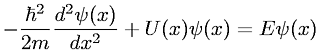Equations > Physics > Modern Physics > Time-independent, one-dimensional Schr

### Time-independent, one-dimensional SchrLatex Code:

MathML Code:

 $-\frac\hslash {}^{2}2m\frac{d}^{2}\psi \left(x\right){\mathrm{dx}}^{2}+U\left(x\right)\psi \left(x\right)=E\psi \left(x\right)$

MathType 5.0: